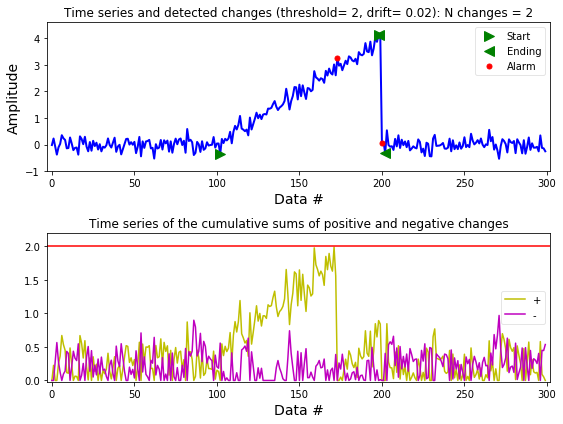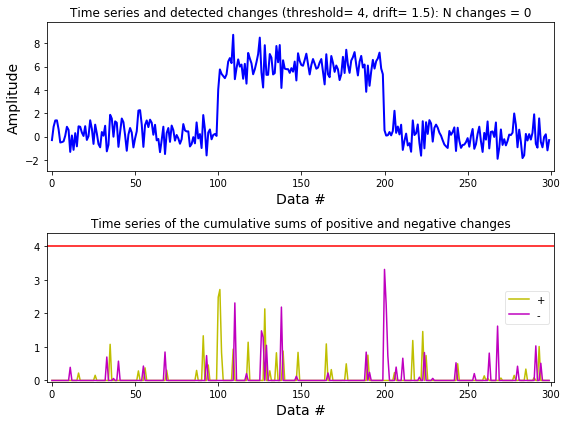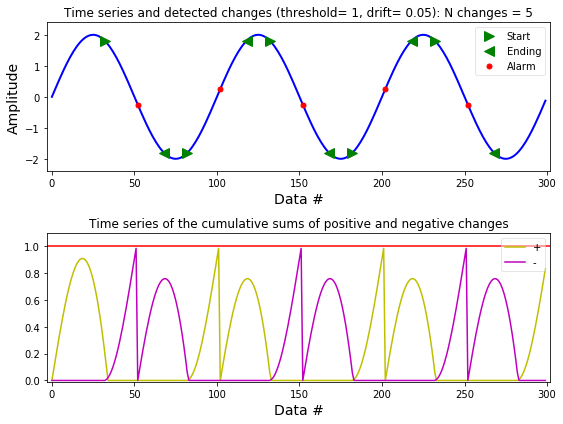Detection of changes using the Cumulative Sum (CUSUM)¶

Marcos Duarte
Laboratory of Biomechanics and Motor Control (http://demotu.org/)
Federal University of ABC, Brazil

Change detection refers to procedures to identify abrupt changes in a phenomenon (Basseville and Nikiforov 1993, Gustafsson 2000). By abrupt change it is meant any difference in relation to previous known data faster than expected of some characteristic of the data such as amplitude, mean, variance, frequency, etc.

The Cumulative sum (CUSUM) algorithm is a classical technique for monitoring change detection. One form of implementing the CUSUM algorithm involves the calculation of the cumulative sum of positive and negative changes ($g_t^+$ and $g_t^-$) in the data ($x$) and comparison to a $threshold$. When this threshold is exceeded a change is detected ($t_{alarm}$) and the cumulative sum restarts from zero. To avoid the detection of a change in absence of an actual change or a slow drift, this algorithm also depends on a parameter $drift$ for drift correction. This form of the CUSUM algorithm is given by:

$$\begin{array}{l l} \left\{ \begin{array}{l l} s[t] = x[t] - x[t-1] \\ g^+[t] = max\left(g^+[t-1] + s[t]-drift,\; 0\right) \\ g^-[t] = max\left(g^-[t-1] - s[t]-drift,\; 0\right) \end{array} \right. \\ \\ \; if \;\;\; g^+[t] > threshold \;\;\; or \;\;\; g^-[t] > threshold: \\ \\ \left\{ \begin{array}{l l} t_{alarm}=t \\ g^+[t] = 0 \\ g^-[t] = 0 \end{array} \right. \end{array}$$

There are different implementations of the CUSUM algorithm; for example, the term for the sum of the last elements ($s[t]$ above) can have a longer history (with filtering), it can be normalized by removing the data mean and then divided by the data variance), or this sum term can be squared for detecting both variance and parameter changes, etc.

For the CUSUM algorithm to work properly, it depends on tuning the parameters $h$ and $v$ to what is meant by a change in the data. According to Gustafsson (2000), this tuning can be performed following these steps:

• Start with a very large $threshold$.
• Choose $drift$ to one half of the expected change, or adjust $drift$ such that $g$ = 0 more than 50% of the time.
• Then set the $threshold$ so the required number of false alarms (this can be done automatically) or delay for detection is obtained.
• If faster detection is sought, try to decrease $drift$.
• If fewer false alarms are wanted, try to increase $drift$.
• If there is a subset of the change times that does not make sense, try to increase $drift$.

The function detect_cusum.py (code at the end of this text) implements the CUSUM algorithm and a procedure to calculate the ending of the detected change. The function signature is:

ta, tai, taf, amp = detect_cusum(x, threshold=1, drift=0, ending=False, show=True, ax=None)

Let's see how to use detect_cusum.py; first let's import the necessary Python libraries and configure the environment:

In :
import numpy as np
%matplotlib inline
import matplotlib.pyplot as plt
import sys
sys.path.insert(1, r'./../functions')  # add to pythonpath
from detect_cusum import detect_cusum

Running the function examples:

In :
>>> x = np.random.randn(300)/5
>>> x[100:200] += np.arange(0, 4, 4/100)
>>> ta, tai, taf, amp = detect_cusum(x, 2, .02, True, True)

>>> x = np.random.randn(300)
>>> x[100:200] += 6
>>> detect_cusum(x, 4, 1.5, True, True)

>>> x = 2*np.sin(2*np.pi*np.arange(0, 3, .01))
>>> ta, tai, taf, amp = detect_cusum(x, 1, .05, True, True)Function performance¶

Here is a poor test of the detect_cusum.py performance:

In :
x = np.random.randn(10000)
x[400:600] += 6
print('Detection of onset (data size = %d):' %x.size)
%timeit detect_cusum(x, 4, 1.5, True, False)
Detection of onset (data size = 10000):
38 ms ± 141 µs per loop (mean ± std. dev. of 7 runs, 10 loops each)

Function detect_cusum.py¶

In [ ]:
"""Cumulative sum algorithm (CUSUM) to detect abrupt changes in data."""

from __future__ import division, print_function
import numpy as np

__author__ = 'Marcos Duarte, https://github.com/demotu/BMC'
__version__ = "1.0.4"

def detect_cusum(x, threshold=1, drift=0, ending=False, show=True, ax=None):
"""Cumulative sum algorithm (CUSUM) to detect abrupt changes in data.

Parameters
----------
x : 1D array_like
data.
threshold : positive number, optional (default = 1)
amplitude threshold for the change in the data.
drift : positive number, optional (default = 0)
drift term that prevents any change in the absence of change.
ending : bool, optional (default = False)
True (1) to estimate when the change ends; False (0) otherwise.
show : bool, optional (default = True)
True (1) plots data in matplotlib figure, False (0) don't plot.
ax : a matplotlib.axes.Axes instance, optional (default = None).

Returns
-------
ta : 1D array_like [indi, indf], int
alarm time (index of when the change was detected).
tai : 1D array_like, int
index of when the change started.
taf : 1D array_like, int
index of when the change ended (if ending is True).
amp : 1D array_like, float
amplitude of changes (if ending is True).

Notes
-----
Tuning of the CUSUM algorithm according to Gustafsson (2000)_:
Start with a very large threshold.
Choose drift to one half of the expected change, or adjust drift such
that g = 0 more than 50% of the time.
Then set the threshold so the required number of false alarms (this can
be done automatically) or delay for detection is obtained.
If faster detection is sought, try to decrease drift.
If fewer false alarms are wanted, try to increase drift.
If there is a subset of the change times that does not make sense,
try to increase drift.

Note that by default repeated sequential changes, i.e., changes that have
the same beginning (tai) are not deleted because the changes were
detected by the alarm (ta) at different instants. This is how the
classical CUSUM algorithm operates.

If you want to delete the repeated sequential changes and keep only the
beginning of the first sequential change, set the parameter ending to
True. In this case, the index of the ending of the change (taf) and the
amplitude of the change (or of the total amplitude for a repeated
sequential change) are calculated and only the first change of the repeated
sequential changes is kept. In this case, it is likely that ta, tai,
and taf will have less values than when ending was set to False.

See this IPython Notebook _.

References
----------
..  Gustafsson (2000) Adaptive Filtering and Change Detection.
..  http://nbviewer.ipython.org/github/demotu/BMC/blob/master/notebooks/DetectCUSUM.ipynb

Examples
--------
>>> from detect_cusum import detect_cusum
>>> x = np.random.randn(300)/5
>>> x[100:200] += np.arange(0, 4, 4/100)
>>> ta, tai, taf, amp = detect_cusum(x, 2, .02, True, True)

>>> x = np.random.randn(300)
>>> x[100:200] += 6
>>> detect_cusum(x, 4, 1.5, True, True)

>>> x = 2*np.sin(2*np.pi*np.arange(0, 3, .01))
>>> ta, tai, taf, amp = detect_cusum(x, 1, .05, True, True)
"""

x = np.atleast_1d(x).astype('float64')
gp, gn = np.zeros(x.size), np.zeros(x.size)
ta, tai, taf = np.array([[], [], []], dtype=int)
tap, tan = 0, 0
amp = np.array([])
# Find changes (online form)
for i in range(1, x.size):
s = x[i] - x[i-1]
gp[i] = gp[i-1] + s - drift  # cumulative sum for + change
gn[i] = gn[i-1] - s - drift  # cumulative sum for - change
if gp[i] < 0:
gp[i], tap = 0, i
if gn[i] < 0:
gn[i], tan = 0, i
if gp[i] > threshold or gn[i] > threshold:  # change detected!
ta = np.append(ta, i)    # alarm index
tai = np.append(tai, tap if gp[i] > threshold else tan)  # start
gp[i], gn[i] = 0, 0      # reset alarm
# THE CLASSICAL CUSUM ALGORITHM ENDS HERE

# Estimation of when the change ends (offline form)
if tai.size and ending:
_, tai2, _, _ = detect_cusum(x[::-1], threshold, drift, show=False)
taf = x.size - tai2[::-1] - 1
# Eliminate repeated changes, changes that have the same beginning
tai, ind = np.unique(tai, return_index=True)
ta = ta[ind]
# taf = np.unique(taf, return_index=False)  # corect later
if tai.size != taf.size:
if tai.size < taf.size:
taf = taf[[np.argmax(taf >= i) for i in ta]]
else:
ind = [np.argmax(i >= ta[::-1])-1 for i in taf]
ta = ta[ind]
tai = tai[ind]
# Delete intercalated changes (the ending of the change is after
# the beginning of the next change)
ind = taf[:-1] - tai[1:] > 0
if ind.any():
ta = ta[~np.append(False, ind)]
tai = tai[~np.append(False, ind)]
taf = taf[~np.append(ind, False)]
# Amplitude of changes
amp = x[taf] - x[tai]

if show:
_plot(x, threshold, drift, ending, ax, ta, tai, taf, gp, gn)

return ta, tai, taf, amp

def _plot(x, threshold, drift, ending, ax, ta, tai, taf, gp, gn):
"""Plot results of the detect_cusum function, see its help."""

try:
import matplotlib.pyplot as plt
except ImportError:
print('matplotlib is not available.')
else:
if ax is None:
_, (ax1, ax2) = plt.subplots(2, 1, figsize=(8, 6))

t = range(x.size)
ax1.plot(t, x, 'b-', lw=2)
if len(ta):
ax1.plot(tai, x[tai], '>', mfc='g', mec='g', ms=10,
label='Start')
if ending:
ax1.plot(taf, x[taf], '<', mfc='g', mec='g', ms=10,
label='Ending')
ax1.plot(ta, x[ta], 'o', mfc='r', mec='r', mew=1, ms=5,
label='Alarm')
ax1.legend(loc='best', framealpha=.5, numpoints=1)
ax1.set_xlim(-.01*x.size, x.size*1.01-1)
ax1.set_xlabel('Data #', fontsize=14)
ax1.set_ylabel('Amplitude', fontsize=14)
ymin, ymax = x[np.isfinite(x)].min(), x[np.isfinite(x)].max()
yrange = ymax - ymin if ymax > ymin else 1
ax1.set_ylim(ymin - 0.1*yrange, ymax + 0.1*yrange)
ax1.set_title('Time series and detected changes ' +
'(threshold= %.3g, drift= %.3g): N changes = %d'
% (threshold, drift, len(tai)))
ax2.plot(t, gp, 'y-', label='+')
ax2.plot(t, gn, 'm-', label='-')
ax2.set_xlim(-.01*x.size, x.size*1.01-1)
ax2.set_xlabel('Data #', fontsize=14)
ax2.set_ylim(-0.01*threshold, 1.1*threshold)
ax2.axhline(threshold, color='r')
ax1.set_ylabel('Amplitude', fontsize=14)
ax2.set_title('Time series of the cumulative sums of ' +
'positive and negative changes')
ax2.legend(loc='best', framealpha=.5, numpoints=1)
plt.tight_layout()
plt.show()